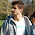## Monday, August 1, 2011

### Python Padding with PKCS7

Here's the definition of PKCS7 padding (from RFC 2315):

``````RFC 2315, section 10.3, note #2:
2.   Some content-encryption algorithms assume the
input length is a multiple of k octets, where k > 1, and
let the application define a method for handling inputs
whose lengths are not a multiple of k octets. For such
algorithms, the method shall be to pad the input at the
trailing end with k - (l mod k) octets all having value k -
(l mod k), where l is the length of the input. In other
words, the input is padded at the trailing end with one of
the following strings:

01 -- if l mod k = k-1
02 02 -- if l mod k = k-2
.
.
.
k k ... k k -- if l mod k = 0

The padding can be removed unambiguously since all input is
padded and no padding string is a suffix of another. This
padding method is well-defined if and only if k < 256;
methods for larger k are an open issue for further study.
``````

And here's how to implement it in python:

```class PKCS7Encoder():
"""
Technique for padding a string as defined in RFC 2315, section 10.3,
note #2
"""
class InvalidBlockSizeError(Exception):
"""Raised for invalid block sizes"""
pass

def __init__(self, block_size=16):
if block_size < 2 or block_size > 255:
raise PKCS7Encoder.InvalidBlockSizeError('The block size must be ' \
'between 2 and 255, inclusive')
self.block_size = block_size

def encode(self, text):
text_length = len(text)
amount_to_pad = self.block_size - (text_length % self.block_size)
if amount_to_pad == 0:
amount_to_pad = self.block_size
pad = chr(amount_to_pad)
return text + pad * amount_to_pad

def decode(self, text):
pad = ord(text[-1])
return text[:-pad]

```

Example use:
```>>> # basic use
>>> encoder = PKCS7Encoder()
>>> padded_value = encoder.encode('hi')
>>> padded_value
'hi\x0e\x0e\x0e\x0e\x0e\x0e\x0e\x0e\x0e\x0e\x0e\x0e\x0e\x0e'
>>> len(padded_value)
16
>>> encoder.decode(padded_value)
'hi'

>>> # empty string
>>> padded_value = encoder.encode('')
>>> padded_value
'\x10\x10\x10\x10\x10\x10\x10\x10\x10\x10\x10\x10\x10\x10\x10\x10'
>>> len(padded_value)
16
>>> encoder.decode(padded_value)
''

>>> # string that is longer than a single block
>>> padded_value = encoder.encode('this string is long enough to span blocks')
>>> padded_value
'this string is long enough to span blocks\x07\x07\x07\x07\x07\x07\x07'
>>> len(padded_value)
48
>>> len(padded_value) % 16
0
>>> encoder.decode(padded_value)
'this string is long enough to span blocks'

>>> # using the max block size
>>> encoder = PKCS7Encoder(255)
>>> padded_value = encoder.encode('hi')
>>> len(padded_value)
255
>>> encoder.decode(padded_value)
'hi'
```

#### 3 comments:

1.Please fix your code, it pads with integer byte blocks instead of HEX as specified in RFC.

def encode:
...
pad = unhexlify('%02x' % amount_to_pad)
...
def decode:
...
pad = int(hexlify(text[-1]), 16)
...

2.Agree with Bojan comment. Compatible code with RFC:

def encode(self, text):
text_length = len(text)
amount_to_pad = self.block_size - (text_length % self.block_size)
if amount_to_pad == 0:
amount_to_pad = self.block_size
#pad = unhexlify('%02d' % amount_to_pad)
pad = chr(amount_to_pad)
return text + pad * amount_to_pad

def decode(self, text):
#pad = int(hexlify(text[-1]))
pad = ord(text[-1])
return text[:-pad]

3.Thank you, I have updated the code and provided some examples.